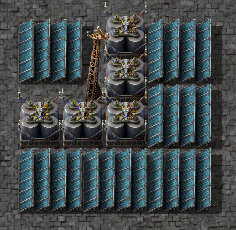# Power production

Electricity has to be produced before it can be transferred to consumers over the electric system. There are multiple methods to produce electricity:

## Steam engine power

Each steam engine needs 0.5 boilers when running at full capacity. One offshore pump can supply 20 boilers and 40 steam engines.

The above ratio can be calculated from information available in-game: One boiler consumes 1.8MW of fuel and produces energy stored in steam at 100% efficiency. One steam engine consumes 900kW of energy stored in steam, so each boiler can supply 2 steam engines: `1.8MW ÷ 0.9MW = 2`. One steam engine consumes 30 steam per second, and one offshore pump produces 1200 water per second, so each offshore pump produces enough water to supply 40 steam engines: `1200 unit/s ÷ 30 units/s = 40`. The number of boilers can be derived from the number of steam engines: `40 ÷ 2 = 20`. This produces the 1:20:40 ratio.

## Solar panels and accumulators

### Optimal ratio

The optimal ratio is 0.84 (21:25) accumulators per solar panel, and 23.8 solar panels per megawatt required by your factory (this ratio accounts for solar panels needed to charge the accumulators). This means that you need 1.428 MW of production (of solar panels) and 100MJ of storage to provide 1 MW of power over one day-night cycle.

A "close enough" ratio is 20:24:1 accumulators to solar panels to megawatts required (for example, a factory requiring 10 MW can be approximately entirely powered, day and night, by 200 accumulators and 240 solar panels - this approximation differs from optimal only in that it calls for 20 extra solar panels, which is negligible but remember that the difference between the "close enough" ratio and the optimal ratio increases as you add more solar panels).

This is taken from Accumulator / Solar Panel Ratio (which calculates this in an impressive mathematical way!) and another post in that thread (which calculates the solar panel to megawatt ratio in a different way).A small 9x9 setup demonstrating the 20:24 "close enough" ratio above.

### Calculations

The optimal ratio of accumulators per solar panel relies on many values in the game. These include the power generation of a solar panel, the energy storage of an accumulator, the length of a day, and the length of a night. There are also times between day and night called dusk and dawn which complicate the calculations. In vanilla factorio, without mods which change any of these values, the optimal ratio will be the same. This ratio is

```Accumulators / Solar_panels =
(day + dawn) × (night + dawn × (day + dawn) / game_day) / game_day
× Solar_power / Accumulator_energy```

which, given the default time lengths of: day = 12500/60 s; dawn or dusk = 5000/60 s; night = 2500/60 s, and the default: Solar_power = 60 kW; Accumulator_energy = 5 MJ = 5000 kJ, gives the optimal ratio of 0.84 accumulators per solar panel. If the player uses mods which change the power generation of solar panels, or the energy storage of accumulators, but not the length of days, a simplified version of this equation can be used.

`Accumulators / Solar_panels = 70 s × Solar_power / Accumulator_energy`

This equation could also be used to remember the vanilla optimal ratio given its simplicity. If the only effect the mod has on the game is it changes the total length of one day, without changing the ratio of dusk : day : dawn : night, then the equation can be simplified as

`Accumulators / Solar_panels = 0.002016 /s × game_day `

where game_day is the number of seconds in the game day which is 25000/60 s by default.

## Nuclear power

In general, nuclear power is produced by the following production chain: Uranium ore is mined and processed to uranium-235 and uranium-238, then uranium fuel cells are created from the two. These fuel cells are then burned in a nuclear reactor to create heat. The heat can be used to convert water to steam using a heat exchanger and the steam can be consumed by steam turbines to produce power.

A reactor without neighbor bonus needs 4 heat exchangers so that all its heat gets consumed. For each 100% neighbor bonus, the reactor needs 4 more heat exchangers.

## Ensuring enough energy is produced

Try this checklist before you completely revamp your power source. You may also use this to rectify brownouts/blackouts.

• Did you connect the steam engine to the electric system? If not, a small yellow triangle will flash. To fix, Add some power poles near the steam engines that go to machines needing that power. Any power pole will work.
• Is steam able to reach all steam engines?
• Do your pipes have water? Look at the windows in the pipes, hover over the pipes! Place some pipes or a tank at the end to see if there is really water coming through. If not, ensure all pipes or underground pipes are connected together.
• Is the factory producing enough fuel (coal, solid fuel, uranium fuel cells)?
• Are there enough steam generators (boilers, heat exchangers)?
• Are there enough steam engines/turbines?

See also the applied power math tutorial to answer the question how much coal do I need?